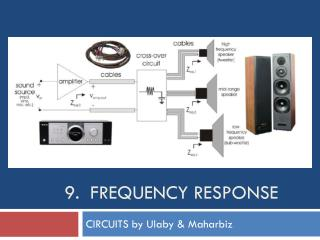DownloadDownload Presentation9. Frequency Response

# 9. Frequency Response

Download Presentation## 9. Frequency Response

- - - - - - - - - - - - - - - - - - - - - - - - - - - E N D - - - - - - - - - - - - - - - - - - - - - - - - - - -
##### Presentation Transcript

1. 9. Frequency Response CIRCUITS by Ulaby & Maharbiz

2. Overview

3. Transfer Function Transfer function of a circuit or system describes the output response to an input excitation as a function of the angular frequency ω. Other Transfer Functions Voltage Gain Magnitude Phase

4. Filters

5. RC Low Pass To determine corner frequency:

6. RC High Pass

7. Filter Terminology Zin1= R + jωL. Im [Zin1] = 0 when ω = 0 Im [Zin2] = 0 requires that ZL = −ZC or, equivalently, ω2 = 1/LC

8. Scaling Scaling is used to configure a prototype version of the intended practical scaled circuit such that in the prototype circuit, element values are on the order of ohms, henrys and farads.

9. dB Scale

10. RL Filter --Magnitude Log scale for ω and dB scale for M

11. RL Filter--Phase Log scale for ω and linear scale for φ(ω)

12. Bode Plots: Straight line approximations Bode Magnitude Slope= 20N dB per decade Bode Phase Slope= 45N degrees per decade 1 decade 1 decade

13. Bode Plots Bode Magnitude Slope= 40dB per decade Bode Phase Slope= 90 degrees per decade

14. Bode Factors

15. Example 9-4: Bode Plots Standard form Numerator: simple zero of second order with corner frequency 5 rad/s Denominator: pole @ origin, and simple pole with corner frequency 50 rad/s

16. Example 9-5: More Bode Plots

17. Example 9-6:Given Bode Plot, Obtain Expression

18. Bandpass RLC Filter

19. Bandpass RLC Filter (cont.) Quality Factor Q: characterizes degree of selectivity of a circuit where Wstoris the maximum energy that can be stored in the circuit at resonance (ω = ω0), and Wdissis the energy dissipated by the circuit during a single period T.

20. Bandpass RLC Filter (cont.) • Derivation of Q Resonant frequency Bandwidth

21. Bandpass Filter

22. Example 9-7: Bandpass Filter Design

23. Highpass Filter Lowpass Filter

24. Bandreject Filter

25. Filter Order

26. Active Filters ̶Lowpass

27. Active Filters ̶Highpass

29. Example 9-10: Third-Order Lowpass Filter

30. Cont.

31. Example 9-11 cont.

32. Cont.

33. Signal Modulation

34. Superheterodyne receiver Frequency of received signal is “down-converted” to a lower intermediate frequency, while retaining the modulation ( which contains the message information) intact

35. Multisim Analysis of RLC Circuit

36. Multisim Analysisof Active Filters

37. Tech Brief 17: Bandwidth and Data Rate Signal-to-noise ratio

38. Tech Brief: Bandwidth and Data Rate Shannon-Hartley Theorem Channel capacity (data rate) in bits/s Bandwidth in Hz Note: A high data rate can be achieved even if the signal power is smaller than the noise, so long as sufficient bandwidth is available.

39. Summary